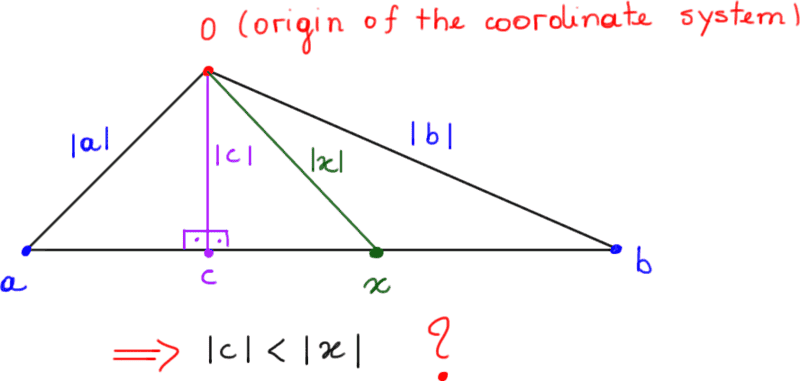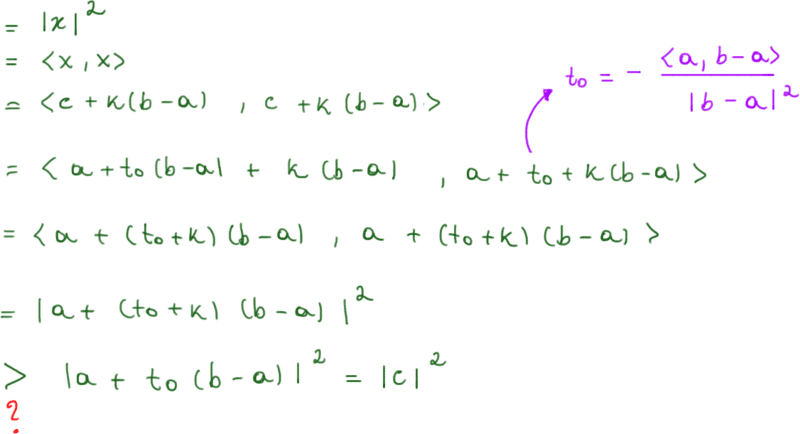# Orthogonal Vectors in Rn Problem

• Onezimo Cardoso
F(t) &= \|a \|^2 + 2 \langle a,(b-a) \rangle t + \| b-a \|^2 t^2 \\&= t^2 \| b-a \|^2 + 2 t \langle a, b-a \rangle + \| a \|^2\end{align*}$$This is a parabola with a minimum at ##t = - \langle a, b-a \rangle / \| b-a \|^2.##In summary, to determine a vector c that lies on a line segment [a,b] and is perpendicular to (b-a), we can use the formula c = a + t_0 ( #### Onezimo Cardoso ## Homework Statement Given ##a\neq b## vectors of ##\mathbb{R}^n##. Determine ##c## which lies in the line segment ##[a,b]=\{a+t(b-a) ; t \in [0,1]\}##, such that ##c \perp (b-a)##. Conclude that for all ##x \in [a,b]##, with ##x\neq c## it is true that ##|c|<|x|##. ## Homework Equations The first part of the question can be solved just using inner product definition. I don't know how the second part can be solved. But I think could be useful the Cauchy-Schawarz inequality ##|<x,y>| \leq |x||y|## or maybe the cosine rule... ## The Attempt at a Solution If we suppose that ##c \in [a,b]## then we can write ##c## as:$$c=a+t_0 (b-a)$$where ##t_0 \in [0,1]##. By the fact that ##c \perp (b-a)## we have:$$<c,(b-a)> \quad = \quad0\Rightarrow \quad <a+t_0 (b-a),(b-a)> \quad = \quad 0\Rightarrow \quad <a,(b-a)>+<t_0 (b-a),(b-a)> \quad =\quad 0\Rightarrow \quad <a,(b-a)>+t_0 |b-a|^2=0\Rightarrow t_0 = - \frac{<a,(b-a)>}{|b-a|^2} $$Then ##c## can be uniquely determined as ##c=a+t_0 (b-a)##, where ##t_0 = - \frac{<a,(b-a)>}{|b-a|^2}##.$$\ldots$$Now we need to prove that if we consider any other ##x \neq c## in the line segment ##[a,b]## then ##|c|<|x|##.#### Attachments Last edited: You can show that ##x = c + k(b-a)## for some ##k \ne 0##. Then calculate ##\langle x,x \rangle##. •Onezimo Cardoso Onezimo Cardoso said: ## Homework Statement Given ##a\neq b## vectors of ##\mathbb{R}^n##. Determine ##c## which lies in the line segment ##[a,b]=\{a+t(b-a) ; t \in [0,1]\}##, such that ##c \perp (b-a)##. Conclude that for all ##x \in [a,b]##, with ##x\neq c## it is true that ##|c|<|x|##. The premise of the question is wrong: if ##a \neq b, \; a,b \in \mathbb{R}^n## it is not necessarily true that ##c \perp (b-a)## for some ##t \in [0,1].## For example, if ##a = (1,1)## and ##b = (2,1)##, we have ##b-a = (1,0)## and ##c = (1,1)+t(1,0) = (1+t,1)##. In order to have ##c \perp (b-a)## we need ##t = -1##, so the"perpendicular" ##c## is not on the segment from ##a## to ##b##; it is outside that segment, but still on the line through ##a## and ##b##. However, if you allow values ##t < 0## and ##t > 1##---in other words, if you allow any ##t \in \mathbb{R}##---then the "minimization" result is true. The easiest way to get that is to minimize ##F(t) \equiv \| c \|^2##, which is a quadratic function of ##t##. Last edited: •Onezimo Cardoso Ray Vickson said: The premise of the question is wrong: if ##a \neq b, \; a,b \in \mathbb{R}^n## it is not necessarily true that ##c \perp (b-a)## for some ##t \in [0,1].## For example, if ##a = (1,1)## and ##b = (2,1)##, we have ##b-a = (1,0)## and ##c = (1,1)+t(1,0) = (1+t,1)##. In order to have ##c \perp (b-a)## we need ##t = -1##, so the"perpendicular" ##c## is not on the segment from ##a## to ##b##; it is outside that segment, but still on the line through ##a## and ##b##. However, if you allow values ##t < 0## and ##t > 1##---in other words, if you allow any ##t \in \mathbb{R}##---then the "minimization" result is true. The easiest way to get that is to minimize ##F(t) \equiv \| c \|^2##, which is a quadratic function of ##t##. Very well noticed Ray Vickson! Ok I can reformulate the question as follow: ## Homework Statement Given ##a\neq b## vectors of ##\mathbb{R}^n##. Determine ##c## which lies in the line ##r## determined by in the line segment ##[a,b]=\{a+t(b-a) ; t \in [0,1]\}##, such that ##c \perp r##. Conclude that for all ##x \in r##, with ##x\neq c## it is true that ##|c|<|x|##. The solution I wrote before can be used in the same way, i.e:$$c = a + t_0 (b-a) \quad where \quad t_0 = - \frac{<a,(b-a)>}{|b-a|^2}$$But regarding the last part, i.e., to prove that ##|x|<|c|## for all ##x \in r##, I have no clue how can I minimize the function ##F(t) \equiv \| x \|^2 \equiv \| a+t(b-a) \|^2##. vela said: You can show that ##x = c + k(b-a)## for some ##k \ne 0##. Then calculate ##\langle x,x \rangle##. In fact vela, we can write any ##x## in the line determined by the line segment ##[a,b]## as ##x = c + k(b-a)##. But follow your tip I stucked at the following red question mark:#### Attachments Don’t substitute for ##c##. Onezimo Cardoso said: Very well noticed Ray Vickson! Ok I can reformulate the question as follow: ## Homework Statement Given ##a\neq b## vectors of ##\mathbb{R}^n##. Determine ##c## which lies in the line ##r## determined by in the line segment ##[a,b]=\{a+t(b-a) ; t \in [0,1]\}##, such that ##c \perp r##. Conclude that for all ##x \in r##, with ##x\neq c## it is true that ##|c|<|x|##. The solution I wrote before can be used in the same way, i.e:$$c = a + t_0 (b-a) \quad where \quad t_0 = - \frac{<a,(b-a)>}{|b-a|^2}

But regarding the last part, i.e., to prove that ##|x|<|c|## for all ##x \in r##, I have no clue how can I minimize the function ##F(t) \equiv \| x \|^2 \equiv \| a+t(b-a) \|^2##.

As I said already, that is a quadratic function of ##t##. Just expand out ##\| a + (b-a)t \|^2##, using the definition of ##\| \cdot \|^2.##